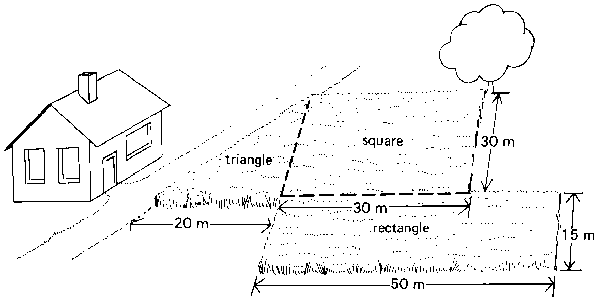## HOW MUCH MATERIAL YOU WILL NEED...

Calculating the amount of material you'll need to complete your project can be trickly. Below we've included a simplified way to explain how to calculate your need. We know this can be confusing and are more then happy to help with your calculation. While all calculations are estimates, this will at least give you an idea of the amount of material you'll need.

###### How Much Material Will You Need?

All coverage amounts are approximate.  Use best judgement when determinig the type and amount of product for your particular application.

To figure out how much material you'll need....

Please use the steps below to find your total area (Square Footage) and calculate an estimation of material you'll need for your project. We've provided this for your convenience, Please don't hesitiate to contact us if you need help.

Square / Rectangle:
Multiply Length times Width to get Square Footage. Then use the Coverage Chart either for depth or rock specifics to get Tons or Yards needed.

Triangle:
Multiply the Base times the Height (or Length times Width) divide in half for Square Footage. Then use the Coverage Chart either for depth or rock specifics to get the Tons or Yards needed.EXAMPLE:
My area is 13 feet by 25 feet.
13' multiplied by 25 feet =  325 sq. ft.
I'm going to put an 1 1/2" rock in this area.  For "Complete Coverage", I'll need to go 3" in depth (two  1 1/2" rocks deep).  I look to my Coverage Chart and 3" depth in Tons (this material sold by the ton)will cover 80 sq.ft. per ton.

So, I'm going to take my Square Footage (325) and divide this number by the approx. coverage (80).  325/80 =  4.06 tons (approx.) of 1 1/2" rock to cover my area.

COVERAGE CHARTS

ONE TON WILL COVER

1" deep ............ 240 sq. feet

2" deep ............ 110 sq. feet

3" deep ............   80 sq. feet

4" deep ............   60 sq. feet

6" deep ............   40 sq. feet

12" deep ...........   20 sq. feet

Based on 2" Depth

NOTE: A number of factors can influence the exact quantities needed for your particular job. You are encouraged to work with us one on one to get a better estimate.

VIEW ADDITIONAL CHARTS BELOW FOR DIFFERENT TYPES OF PRODUCTS

CONVERSIONS:
1 Acre=43,560 sq.ft.
1 Cubic Yard=27 cubic feet
1 Ton=2,000 pounds

Stone / Gravel

Here's what you do:

• 3/8" Rock → divide area by 110

• 1/2"- Rock → divide area by 110

• 3/4" Rock → divide area by 110

• 1 1/2" Rock → divide area by 80

Veneer Moss Rock

• divide area by 25

Flagstone

• divide area by 115

Results will be in TONS

Soil Mixes

*Depends on depth*
Are you topdressing or applying
several inches thick?

Here's what you do:

• 1/4" thick → divide area by 1290

• 1/2" thick → divide area by 640

• 1 inch deep → divide area by 300

• 2 inch's deep → divide area by 120

• 3 inch's deep → divide area by 100

• 4 inch's deep → divide area by 75

• 6 inch's deep → divide area by 50

• 8 inch's deep → divide area by 42

• 10 inch's deep → divide area by 33

• 12 inch's deep → divide area by 25

The results will be in CUBIC YARDS

Playground Mulch - Recommended Depth:

• Equipment Height 6' → use 6" material depth

• Equipment height 7' → use 9" material depth

• Equipment Height 8' → use 10" material depth

• Equipment height 10' → use 12" material depth

Bases and Fill Material

*Depends on depth*
Are you topdressing or applying
several inches thick?

Here's what you do:

• 1 inch deep → divide area by 240

• 2 inch's deep → divide area by 110

• 3 inch's deep → divide area by 80

• 4 inch's deep → divide area by 60

• 6 inch's deep → divide area by 40

• 8 inch's deep → divide area by 33

• 10 inch's deep → divide area by 26

• 12 inch's deep → divide area by 20

The results will be in TONS

This will give you the approx. amount of material you will need for your project.

The results will be in Tons or Yards.

TIPS:

• Most driveways are 10 to 12 feet wide

• Are you filling the WHOLE area?   If there's a large object also filling the area.. account for that.

• When figuring the area of a retaining wall use Length x Height

• If the any of the lengths vary (example: my wall starts off at 2 feet but then tapiers off to zero) use the average (in this example the average would be 1 foot)....  or break the area into different sections.

Having problems?  Do you have something specific?

Give us a call (909) 887-3960 or Email Us

WE CAN HELP!

###### GRAVEL

We are only a phone call away!